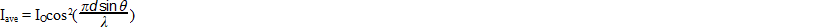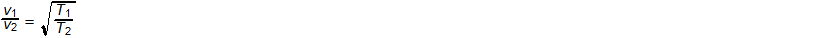### AP Physics 1 Week 8 (July 30 - Aug 05, 2017)

Part 1:  Algebra (Rearrange)

1.  solve for d2. solve for l3. solve for q4.  solve for v25.  solve for T2Part 2:  Algebra (Inverse and Direct Relationships)

6  The load that a wooden beam of given length can support varies jointly as its width and the square of its depth.  If a beam whose width is 4 inches and whose depth is 2 inches can support a load of 880 pounds, find the load the beam can support when turned on its side, that is a width of 2 inches and a depth of 4 inches.  (DBW, p316, #5)

7  The heat developed in a wire carrying a current for a specified time varies jointly as the resistance and the square of the current.  If a current of 8 amperes produces 153.6 calories in a wire whose resistance is 10 ohms, how many calories are produced by a current of 6 amperes in a wire whose resistance is 15 ohms?  (DBW, p316, #6)

8  A surface is now 10 feet from a source of light.  How far from the source would the surface have to be in order to receive twice as much illumination if the intensity varies inversely as the square of the distance from the source of light?  (DBW, p317, #7)

9  When an object moves in a circular path, the centripetal force varies directly as the square of the velocity and inversely as the radius of the circle.  If the force is 64 Newtons when the velocity is 2 meters per second at a radius of 5 meters, find the force when the velocity is 3 meters per second at a radius is 4 meters. (DBW, p317, #8)

10  If a satellite completes a circular orbit in 90 minutes flying 500 miles above the surface of the earth at a rate of 18,000 miles per hour, how long would it take a space capsule flying 300 miles above the earth at a rate of 18,950 miles per hour to complete one orbit?  Consider 4000 miles as the radius of the earth, and use the fact that the period in hours for a satellite to complete a circular orbit varies directly as the radius of orbit and inversely as the orbital velocity.  (DBW, p317, #9)

Part 3: Geometry/Trigonometry
(For Physics, make sure your calculator is set in degrees)

Right Triangle Review:
Pythagorean Theorem (math version) c2 = a2 + b2, (physics version) r2 = x2 + y2
r = hypotenuse of the right triangle
x = horizontal or (east-west) component of the triangle
y = vertical or (north-south) component of the triangle
q = the reference angle always between the x axis and the hypotenuse

horizontal component x = r*cos(q)  vertical component y = r*sin(q)

11  Jim can swim at a rate of 3 miles per hour.  If he heads for a point directly across a river in which the current is 10 miles per hour, by how many degrees does the direction in which he actually swims differ from his intended direction?
-12.  If the river is 32 yards wide, will he make it across before reaching the falls 112 yards downstream?

(1 mile = 1760 yards)  (DBW, p 394, #7)

13   In a military test, a ballistic missile has a target 250 miles east and 280 miles south of its launching site.  How far must it travel to the target?
-14.  If a second missile is sent from the target site to intercept the first missile, at what heading (direction) should it be sent?  (DBW, p 394, #8)

15.  A boy is pulling his sister on a sled.  The rope makes an angle of 38 degrees with the sled.  If the boy is exerting a pull of 30 lbs. on the rope, what force in pounds is being exerted on the sled to pull it forward across the snow?  (DBW, p 397, #1)

Part 4:  Word Problems

16. The speed of a body freely falling from rest is directly proportional to the length of time that it falls.  If a body was falling at 144 ft per second 4.5 seconds after beginning its fall, how fast was it falling 3.75 seconds later? (DBW, p216, #7)

17. A ball which rolls off a penthouse terrace falls 16 feet the first second, 48 feet the next second, and 80 feet the third second.  If it continues to fall in this manner, how far does it fall in the seventh second?  (DBW, p490, #6)

18. A missile fired vertically upward rises to a height of 15,840 feet the first second, then to 15,808 feet the following second, and 15,776 feet the third second.  How many feet does it rise to in the 45th second?  How many feet and in what direction does it move in the ninth minute after it is fired?  (DBW, p490, #7)

19. A rocket averaged a vertical speed of 750 miles per hour during its ascent.  At the peak of its flight path, it released a capsule which made a controlled descent with an average vertical speed of 400 miles per hour.   If the capsule returned 57.5 minutes after the rocket was launched, find the height reached by the rocket.  (DBW, p175, #10)

20. At a rocket launching site a tracker located 1.5 miles from a rocket launch pad saw the first flame of the rocket on the pad 7.33 seconds before he heard the sound associated with the combustion.  A tracker located at another site heard the sound 5.5 seconds after the flame began.  How far was the second tracker from the launch pad?

(DBW, p217, #14)